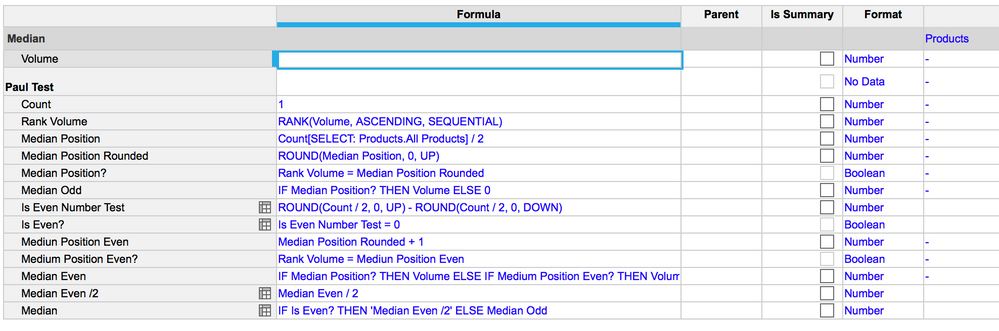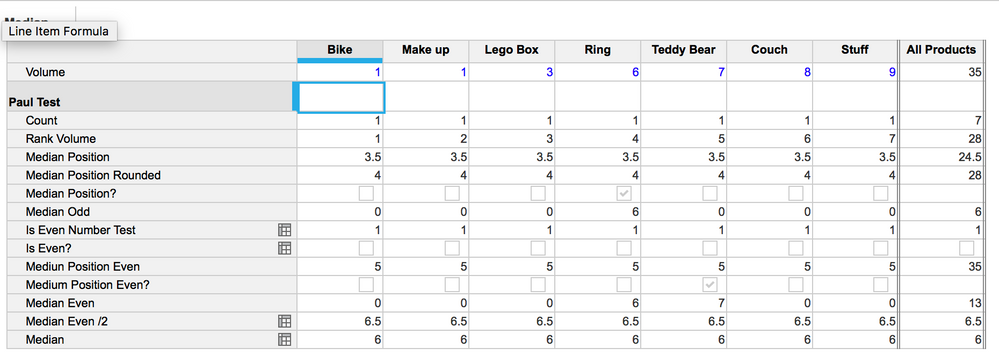# Finding the MedianHi Guys, thanks to all of you that watched the session on Anaplan Live! Apologies for any technical issues you had to sit through.

One thing I said I would post was the problem solving section of Finding the Median. As you are most likely aware by now there is no function in Anaplan for this so you have to work around it using a little bit of logic and other functions.

Credit to Seb McMillan for walking me through this.

1. Use a count (formula is just "1") to calculate the total number of entries.

2. Rank the volume ascending and sequentially

3. Divide the count total by 2

4. Round step 3 "UP"

5. Using a boolean, match the Rank value to the Rounded up figure from step 4.

6. If Statement to connect the boolean to the volume.

Two problems we have so far.

1. Zeros are NOT classed as null and will be counted in the data set.

2. The above will only work if you have an odd number of products/items

So we must continue:

7. We must create an indicator to understand if it is an even number or not. I used this formula "ROUND(Count / 2, 0, UP) - ROUND(Count / 2, 0, DOWN)" this will bring back a zero if it is an even number.

8. Using a boolean, match the formula above to zero

9. We now need to find a secondary position for our median to work alongside the one we created before. In my situation, I have just added +1 to step Step 4 in a new line item.

10. Using a boolean, I now match the new position to the original rank.

11. I bring back the number off both positions using a nested IF statement (nobody shout at me for this please)

12. Divide the previous steps total by 2, to create my Median on an even number of items

13. Lastly I use Step 8 to dictate which answer I use. If even, go get me this number, if odd, get me the original one.

Hope this helps, I have added a screenshot of both the grid and the blueprint to help.Paul